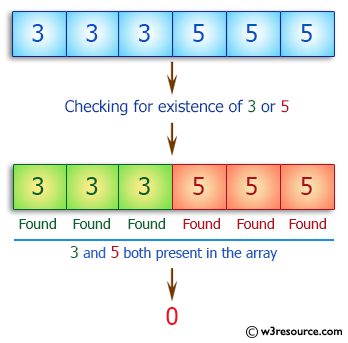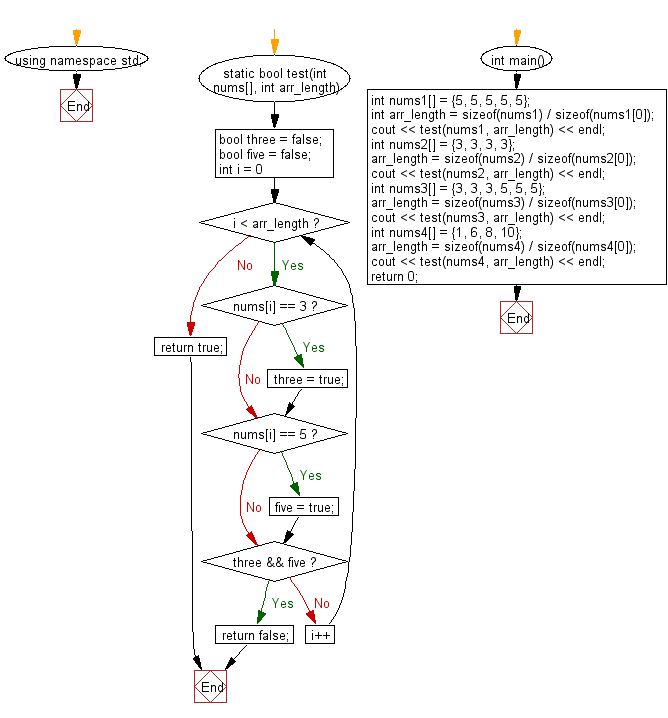﻿ C++ : Check if an array of integers contains no 3 or a 5# C++ Exercises: Check if a given array of integers contains no 3 or a 5

## C++ Basic Algorithm: Exercise-107 with Solution

Write a C++ program to check if a given array of integers contains no 3 or a 5.

Sample Solution:

C++ Code :

``````#include <iostream>
using namespace std;

static bool test(int nums[], int arr_length)
{
bool three = false;
bool five = false;

for (int i = 0; i < arr_length; i++)
{
if (nums[i] == 3) {three = true;}
if (nums[i] == 5) {five  = true;}
if (three && five) return false;
}
return true;
}

int main()
{
int nums1[] = {5, 5, 5, 5, 5};
int arr_length = sizeof(nums1) / sizeof(nums1);
cout << test(nums1, arr_length) << endl;
int nums2[] = {3, 3, 3, 3};
arr_length = sizeof(nums2) / sizeof(nums2);
cout << test(nums2, arr_length) << endl;
int nums3[] = {3, 3, 3, 5, 5, 5};
arr_length = sizeof(nums3) / sizeof(nums3);
cout << test(nums3, arr_length) << endl;
int nums4[] = {1, 6, 8, 10};
arr_length = sizeof(nums4) / sizeof(nums4);
cout << test(nums4, arr_length) << endl;
return 0;
}
``````

Sample Output:

```1
1
0
1
```

Pictorial Presentation:Flowchart:C++ Code Editor:

Contribute your code and comments through Disqus.

What is the difficulty level of this exercise?

﻿